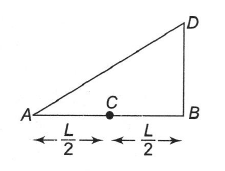A triangular plate of uniform thickness and density is made to rotate about an axis perpendicular to the plane of the paper and (i) passing through A, (ii) passing through B, by the application of some force F at C (mid-point of AB) as shown in the figure. In which case angular acceleration is more?

# A triangular plate of uniform thickness and density is made to rotate about an axis perpendicular to the plane of the paper and (i) passing through A, (ii) passing through B, by the application of some force F at C (mid-point of AB) as shown in the figure. In which case angular acceleration is more?1. A

In case (i)

2. B

In case (ii)

3. C

Both (i) and (ii)

4. D

None of these

Register to Get Free Mock Test and Study Material

+91

Verify OTP Code (required)

### Solution:

We can see that torque in both cases is $\frac{\mathrm{FL}}{2}$. But in case (ii) moment of inertia is less. So angular acceleration is more in case (ii).Register to Get Free Mock Test and Study Material

+91

Verify OTP Code (required)